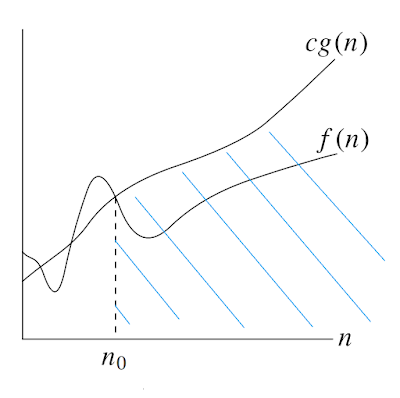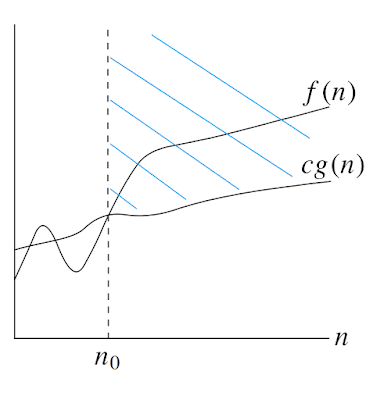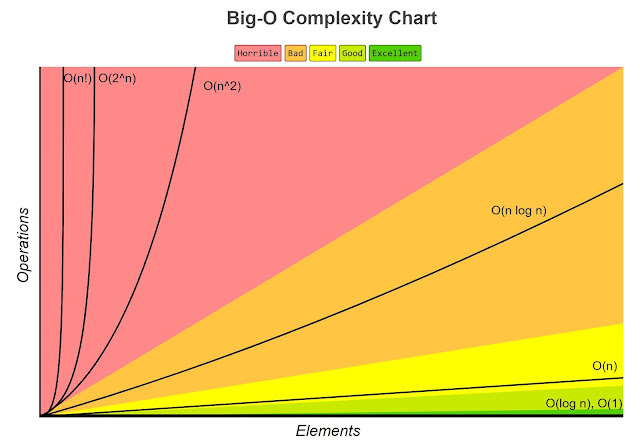Showing posts with label Big-O. Show all posts
Showing posts with label Big-O. Show all posts

## Wednesday, June 5, 2019

### Introduction in Big-O Notation

Big O notation is a mathematical notation that describes the limiting behavior of a function when the argument tends towards a particular value or infinity. It is a member of a family of notations invented by Paul Bachmann, Edmund Landau, and others, collectively called Bachmann-Landau notation or asymptotic notation.

Academics use big O, big Θ (theta), big Ω (omega) to describe runtimes.

#### O (big O)f(n) = O(g(n))
O-notation gives an upper bound for a function to within a constant factor. We write f (n) = O(g(n)) if there are positive constants n0 and c such that to the right of n0, the value of f (n) always lies on or below cg(n).

#### Ω (big omega)f(n) = Ω(g(n))
Ω -notation gives a lower bound for a function to within a constant factor. We write f (n) = Ω (g(n)) if there are positive constants n0 and c such that to the right of n0, the value of f(n) always lies on or above cg(n).

#### Θ (big theta)f(n) = Θ(g(n))
Θ-notation bounds a function to within constant factors. We write f(n) = Θ(g(n)) if there exist positive constants n0, c1, and c2 such that to the right of n0, the value of f(n) always lies between c1g(n) and c2g(n) inclusive.

#### Big O notation in software development industry

In software development industry, people seem to have merge all this concept into one. In software development we use Big O notation to work out how long an algorithm will take to run. This lets us understand how a piece of code will scale. It measures algorithmic efficiency.

Using O-notation, we can often describe the running time of an algorithm merely by inspecting the algorithm’s overall structure. For example, the doubly nested loop structure yields an O(n^2) on the worst-case running time.

### Running time of an algorithm

1. O(log n)
2. O(1)
3. O(n)
4. O(n log n)
5. O(n^2)
6. O(2^n)
7. O(n!)Big-O Complexity Chart (http://www.bigocheatsheet.com/)